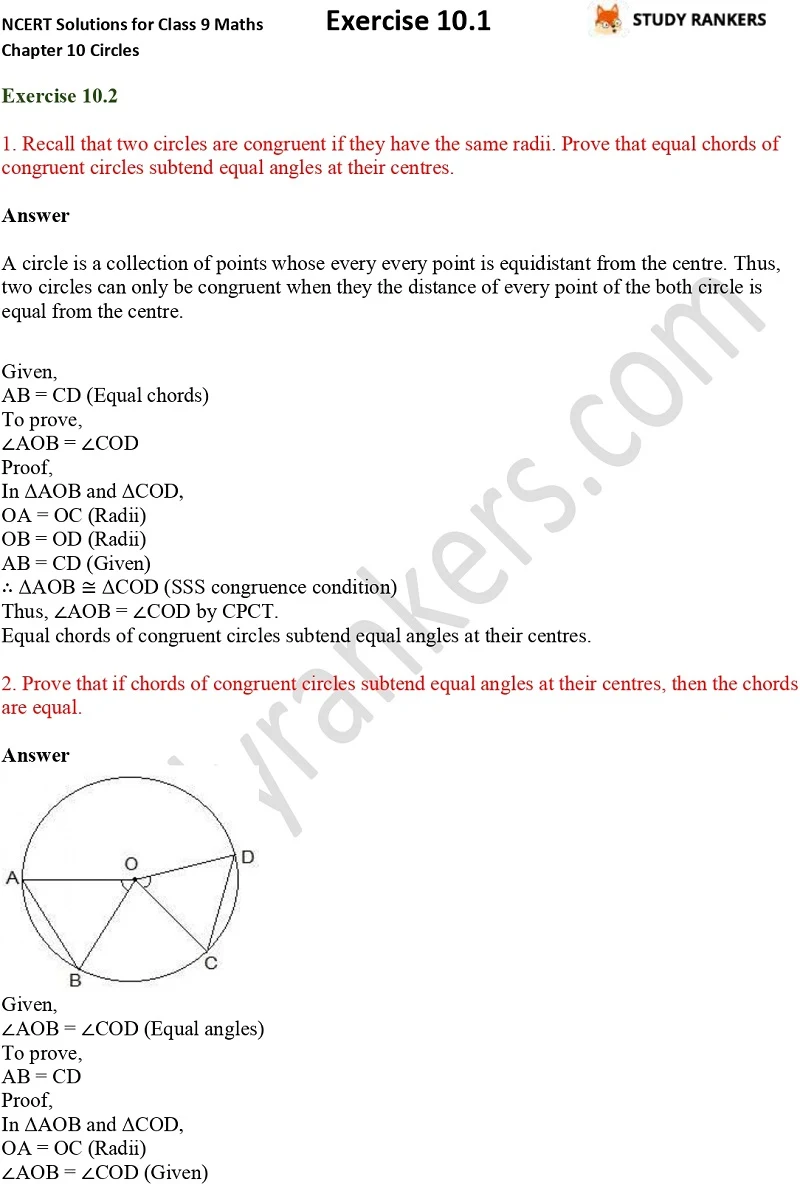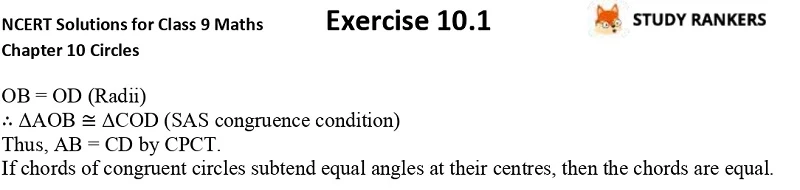## NCERT Solutions for Class 9 Maths Chapter 10 Circles Exercise 10.2

If you're facing any problem in finding the NCERT Solutions of Chapter 10 Circles Exercise 10.2 Class 9 Maths then you can find them here. NCERT Solutions for Class 9 Maths is very valuable guide that will inform about important formulas of the chapter. NCERT questions and answers prepared by Studyrankers experts are detailed so you can understand the concepts easily. By taking help from these solutions, you can complete home assignments on time.

Exercise 10.2 has two questions which are proving that equal chords of congruent circles subtend equal angles at their centres etc.